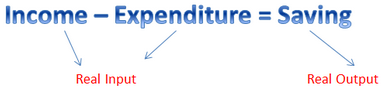Courses

# Algebra of Real Functions Mathematics Notes | EduRev

## Algebra for IIT JAM Mathematics

Created by: Veda Institute

## Mathematics : Algebra of Real Functions Mathematics Notes | EduRev

The document Algebra of Real Functions Mathematics Notes | EduRev is a part of the Mathematics Course Algebra for IIT JAM Mathematics.
All you need of Mathematics at this link: Mathematics

Assume a case where Seema, whose monthly income is Rs. 15,000, spends Rs. 10,000. What will be her saving? Simple! Rs. 5,000. Saving = Income – Expenditure. Here, we see that the input and the output are the real numbers. We can say that real input gives a real output. Here, we will learn Real-valued functions and algebra of real functions. The above case is a representation of real mathematical functions and a case of subtraction in the algebra of real functions.

Real-valued Mathematical Functions
In mathematics, a real-valued function is a function whose values are real numbers. It is a function that maps a real number to each member of its domain. Also, we can say that a real-valued function is a function whose outputs are real numbers i.e., f: R(stands for Real).Algebra of Real Functions
In this section, we will get to know about addition, subtraction, multiplication, and division of real mathematical functions with another.

Let f and g be two real valued functions such that f: X→and g: X→where X ⊂ R. The addition of these two functions (f + g): X→R  is defined by:
(f + g) (x) = f(x) + g(x), for all x ∈ X.

Subtraction of One Real Function from the Other
Let f: X→and g: X→be two real functions where X ⊂ R. The subtraction of these two functions (f – g): X→R  is defined by:
(f – g) (x) = f(x) – g(x), for all x ∈ X.

Multiplication by a Scalar
Let f: X→be a real-valued function and γ be any scalar (real number). Then the product of a real function by a scalar γf: X→is given by:
(γf) (x) = γ f(x), for all x ∈ X.

Multiplication of Two Real Functions
The product of two real functions say, f and g such that f: X→and g: X→R, is given by
(fg) (x) = f(x) g(x), for all x ∈ X.

Division of Two Real Functions
Let f and g be two real-valued functions such that f: X→and g: X→where X ⊂ R. The quotient of these two functions (f  ⁄ g): X→R  is defined by:
(f / g) (x) = f(x) / g(x), for all x ∈ X.
Note: It is also called pointwise multiplication.

Solved Example for You
Problem: Let f(x) = xand g(x) = 3x + 1 and a scalar, γ= 6. Find

1. (f + g) (x)
2. (f – g) (x)
3. (γf) (x)
4. (γg) (x)
5. (fg) (x)
6. (f / g) (x)

Solution: We have,

1. (f + g) (x) = f(x) + g(x) = x+ 3x + 1.
2. (f – g) (x) = f(x) – g(x) = x– (3x + 1) = x– 3x – 1.
3. (γf) (x) = γ f(x) = 6x
4. (γg) (x) = γ g(x) = 6 (3x + 1) = 18x + 6.
5. (fg) (x) = f(x) g(x) = x(3x +1) = 3x4 + x3.
6. (f / g) (x) = f(x) / g(x) = x/ (3x +1), provided x ≠ – 1/3.
Offer running on EduRev: Apply code STAYHOME200 to get INR 200 off on our premium plan EduRev Infinity!

58 docs

,

,

,

,

,

,

,

,

,

,

,

,

,

,

,

,

,

,

,

,

,

;